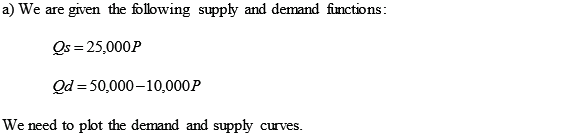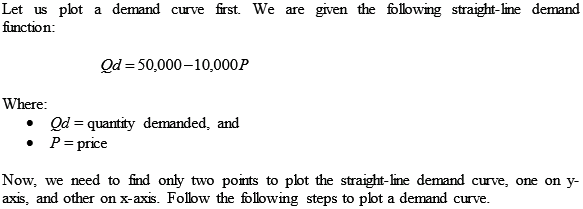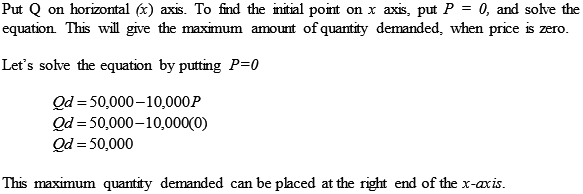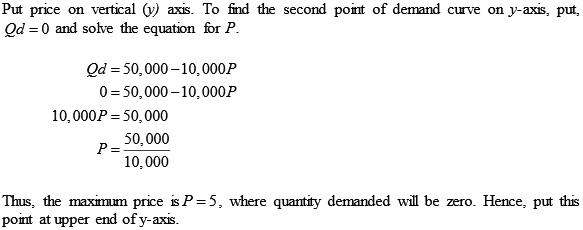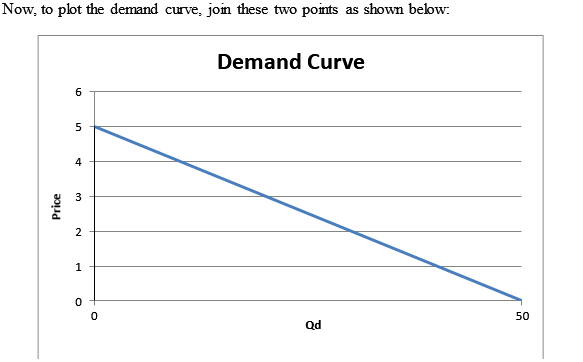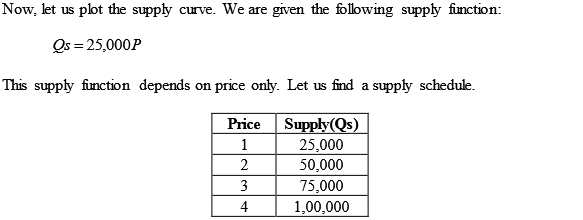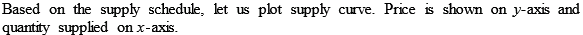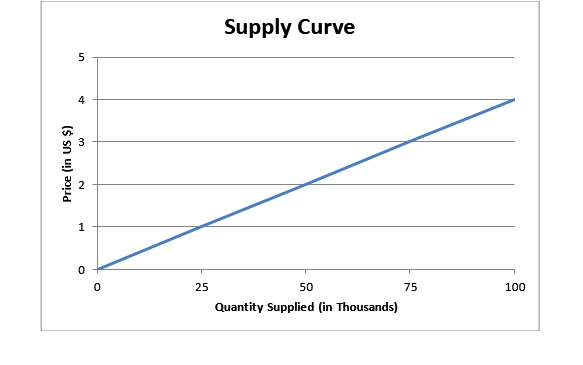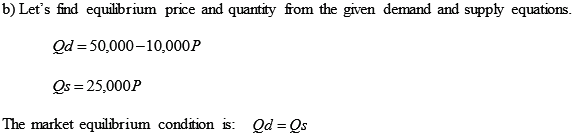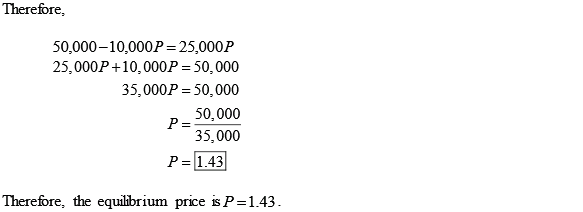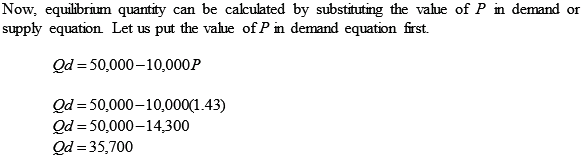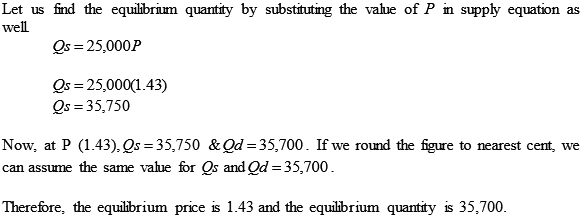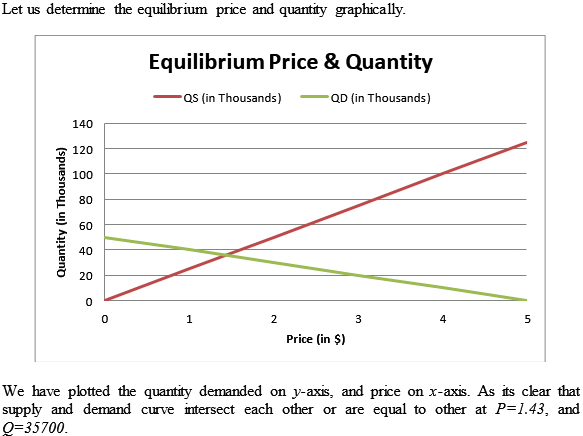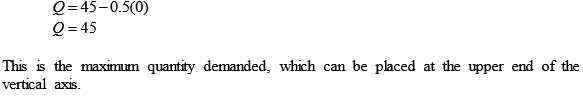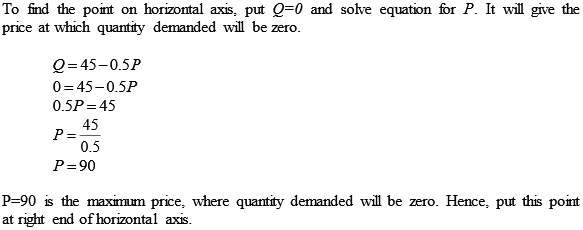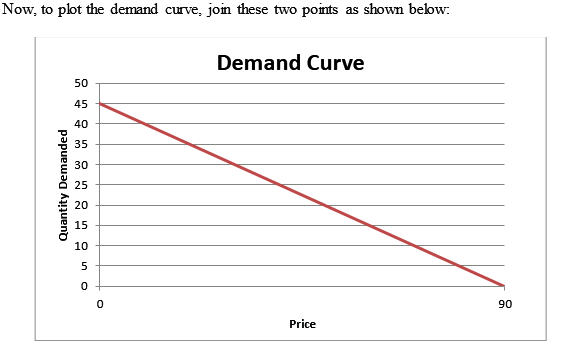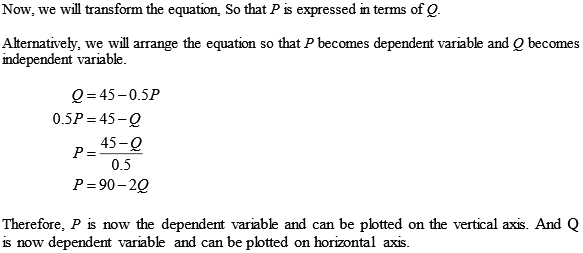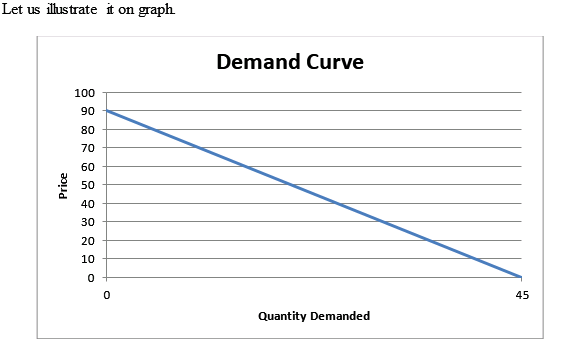# Quiz 3: Supply and Demand

Business

(a) We are given the following demand equation: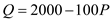Where: • Q is cap sales and • P is price. Now, we need to calculate, how many caps could be sold at \$12 each. Then again, we need to calculate the quantity (Q) at price (P) of \$12 each. To find the quantity (Q), simply substitute P = 12 in to the demand equation.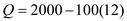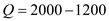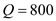Thus, 800 caps could be sold at \$12 each. (b) We are given the following demand equation:Where: • Q is cap sales and • P is price. Now, we need to calculate, what should be the price in order for the company to sell 1000 caps. To find the price, simply substitute Q = 1000 in to the demand equation, and solve the equation.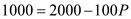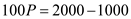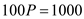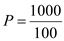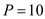Thus, the price should be \$10 in order for the company to sell 1000 caps. (c) We are given the following demand equation:Where Q is cap sales and P is price. Now, we need to calculate the price at which cap sales would equal zero. It can be calculated by simply substituting Q = 0 in to the demand equation.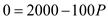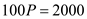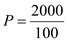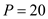Thus, cap sales would equal to zero at price of \$20 each.

Demand : Demand is the quantities of a good or service that a consumer has the willingness (preference) and ability (income) to buy at various prices and in a given frame of time, keeping other determinants of demand constant. So, in economics, demand is defined as something that the consumer is both able and willing to buy. This means the desire to own something alone cannot be considered as demand the consumer must have the financial ability to pay for it too. Demand for a good or service is influenced by various factors other than price, like consumer's income, age, sex, habits, tastes, preferences, prices of substitute goods etc. Market demand is the sum total of the quantity demanded of a product or service by all the consumers in the market at a given price and at a given period. It is the aggregate of all the individual demands. It is graphically represented by downward sloping market demand curve, which is derived by combining the individual demands of all buyers of that product in the market. Downward sloping or negatively sloped demand curve depicts the law of demand, which shows that buyer's ability and willingness to buy is inversely related to its price. Buyers will buy more at lower price, and less at higher price. Quantity Demanded : Quantity demanded is the specific quantity desired or demanded by the consumer at a particular given price, and at given point of time. The quantity demanded depends solely on the price level in the market. Difference between Demand and Quantity demanded There is a huge difference between the term demand and quantity demanded. Demand is a concept, while quantity demanded is a number.The term demand represents the entire relationship between various levels of quantity demanded of a good and all possible prices charged for that good. While quantity demanded represents the specific quantity desired or demanded at a particular given price. The term demand is associated with the whole demand curve, which depicts various levels of quantity demanded and prices. On the other hand, quantity demanded is just a point on the whole demand curve. The difference between the terms demand and quantity demanded can easily be understood by explaining the respective changes and the sources of changes in both these terms. Change in demand is caused by changes in factors other than price. A change in these non-price factors (consumer's income, tastes, preferences, prices of substitute and complementary goods etc) changes the demand in a way, that it shifts the entire demand curve either upward or downward. On the other hand a change in quantity demanded is an outcome of change in price only. When price changes quantity demanded changes accordingly, following the law of demand. As the demand curve represents the relationship of quantity demanded and price only, therefore a change in quantity demanded because of change in price causes just movement from one point to another along the same demand curve. So, when there is a change in price, only the quantity demanded changes, but demand remains the same. We can conclude that change in quantity demanded is just a movement along the demand curve and it is solely caused by changes in price. While a change in demand involves the entire shift of demand curve which occurs because of changes in non price determinants of demand. Supply : Supply stands for the different amount of quantities of a product that a seller is ready to sell at various prices and in a given frame of time, keeping all other non-price factors constant. The various non-price factors that affect supply are costs, technology, number of sellers, prices of other goods or services offered by seller, future expectations, weather conditions etc. Supply depicts the entire relationship between different levels of prices and the corresponding different quantities of the product supplied at each price level. The law of supply states that there is a positive relationship between price and quantity supplied. As the price increases, quantity supplied increases and vice versa. This relationship is exhibited with the help of supply curve, which has a positive or upward slope, showing the direct relationship between price and quantity supplied. Quantity Supplied : Quantity supplied is the amount of goods or services that is supplied by the producer at a specific market price, and at a given point of time. The quantity supplied depends on the price level at a given time in the market. It is just a point on the entire supply curve. Difference between Supply and Quantity supplied Supply is a broad concept, while quantity supplied is a narrow concept.The basic difference between supply and quantity supplied is that supply represents various possible relationships between price and quantity along a supply curve. While quantity supplied is just a point on that curve which represents the relationship between a particular price and a resultant particular quantity supplied. Another major difference is the effect of change in both supply and quantity supplied on the supply curve. A change in supply causes an entire shift in supply curve. This is because of the affect of non-price determinants on supply. For example, a fall in unit cost of production will result in an increase in supply, and the supply curve will shift towards right. It will change the whole range of prices and quantities. On the other hand a change in quantity supplied depicts just a movement along the same supply curve from one point to another. For example, if the price of the product rises, the quantity supplied will accordingly increase and there will be an upward movement from one point to another along the same supply curve. Therefore, we can conclude that, supply is the "entire supply curve", which shifts or relocate because of changes in non-price factors. While quantity supplied is just a point on a supply curve, which moves from one point to another because of changes in price only.